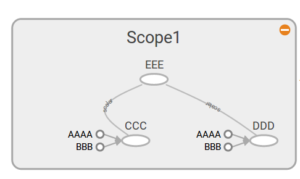# Tensorflow 3: Tensorboard Basics

Expanding from my previous post:

I will create a new jupyter notebook called 3_TensorboardBasics.ipynb.  All my code can be found on github.

#### Tensorboard

Tensorflow comes with a program to help visualize the computational graph called Tensorboard.  Tensorboard is very useful and powerful and ill show you how to get up and running with it.

To see what this is we will create a more complicated example.

```#New graph
a=tf.constant(4)
b=tf.constant(3)
d=tf.multiply(a,b)
e=tf.multiply(c,d)

with tf.Session() as session:
result = session.run(e)
print(result)

#create tensorboard
writer = tf.summary.FileWriter('./my_graph', graph=tf.get_default_graph())
```

This introduces two new Tensorflow commmands (add, multiply) that work as expected.

The interesting line is:

`tf.summary.FileWriter('./my_graph', graph=session.graph)`

This creates a new directory called my_graph in the jupyter notebook directory.To view the contents of this file we need to run a Tensorboard.

run the following command.You access the Tensorboard via a webrowser at http://127.0.0.1:6006.  Click on the Graphs tab up the top to view the graph you just made.#### Naming

Looking at above its very hard to tell what is what.  Luckily Tensorflow allows you to name the parts of your graph.

I will name each part and also place them all into a namescope which groups items together.

```#New graph
with tf.name_scope("Scope1"):
a=tf.constant(4,name="AAAA")
b=tf.constant(3,name="BBB")
d=tf.multiply(a,b,name="DDD")
e=tf.multiply(c,d,name="EEE")

with tf.Session() as session:
result = session.run(e)
print(result)

#create tensorboard
writer = tf.summary.FileWriter('./my_graph', graph=tf.get_default_graph())```

Now the graph appears as:and when clicked expands to show the inner parts also named.Note when using Tensorboard with jupyter it will often cache results so you may need to restart both jupyter, tensorboard and delete the log file.

#### Script

I like to use the following script when executing native python code.

```#!/bin/bash
echo Hello
source ~/tensorflow/bin/activate      # bash, sh, ksh, or zsh
python 2.py
tensorboard --logdir="my_graph"```

Which is executed by running:  ./RUNNERThis will change to your virtual environment, execute the python script and run Tensorboard.

Move onto the next item.